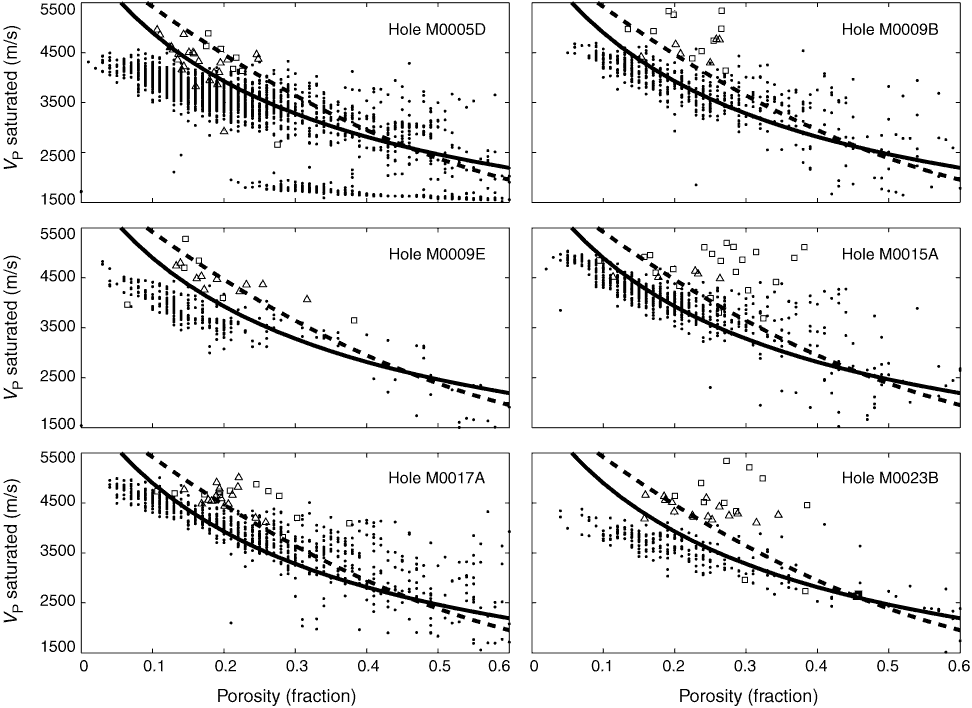Figure F6. Cross-plots of porosity vs. P-wave velocity derived from core-log data. Triangles = discrete laboratory measurements, rectangles = discrete shipboard measurements. Solid lines = time-average equation for solid-phase velocities of 6.5 km/s (calcite), dashed lines = Raymer equation for solid-phase velocities of 6.5 km/s (calcite).